# Control of reaction-diffusion under state constraints - Numerical exploration of controls

Author: - 04 April 2020

Download Code
##### Control of reaction-diffusion

This is a post in a collection of several on control of reaction-diffusion under state constraints

In this tutorial we will observe different phenomena that arise in the control with state-constraints and moreover we will observe different ways to approach a desired target.

# Observation of several phenomena

In the simulations below, we will consider the following optimal control problem:

In Figure 1, the initial state was $u_0=1$ and we can observe how the solution has been successfully driven to the target $u_1=0.33$. For this length of the domain there is no barrier (see Barriers)), for this reason the optimal solution can achieve the target.

The trajectory of the control can also be understood from the theoretical understanding of the controllability under state constraints.

In the first phase, the control takes values that are below the target $u_1=0.33$. This behavior is necessary because for this length of the domain there exist nontrivial solutions with boundary value $0.33$ that are between $0$ and $1$.

These nontrivial solutions do not constitute an intrinsic obstruction since we know that the equation is controllable for this length of the domain (see [1,3] and Staircase post and Barriers post). However, the strategy of setting boundary equal to $0.33$ for a long time and then doing a local control will not work.

The second oscillatory phase has been already observed in the unconstrained case (see ).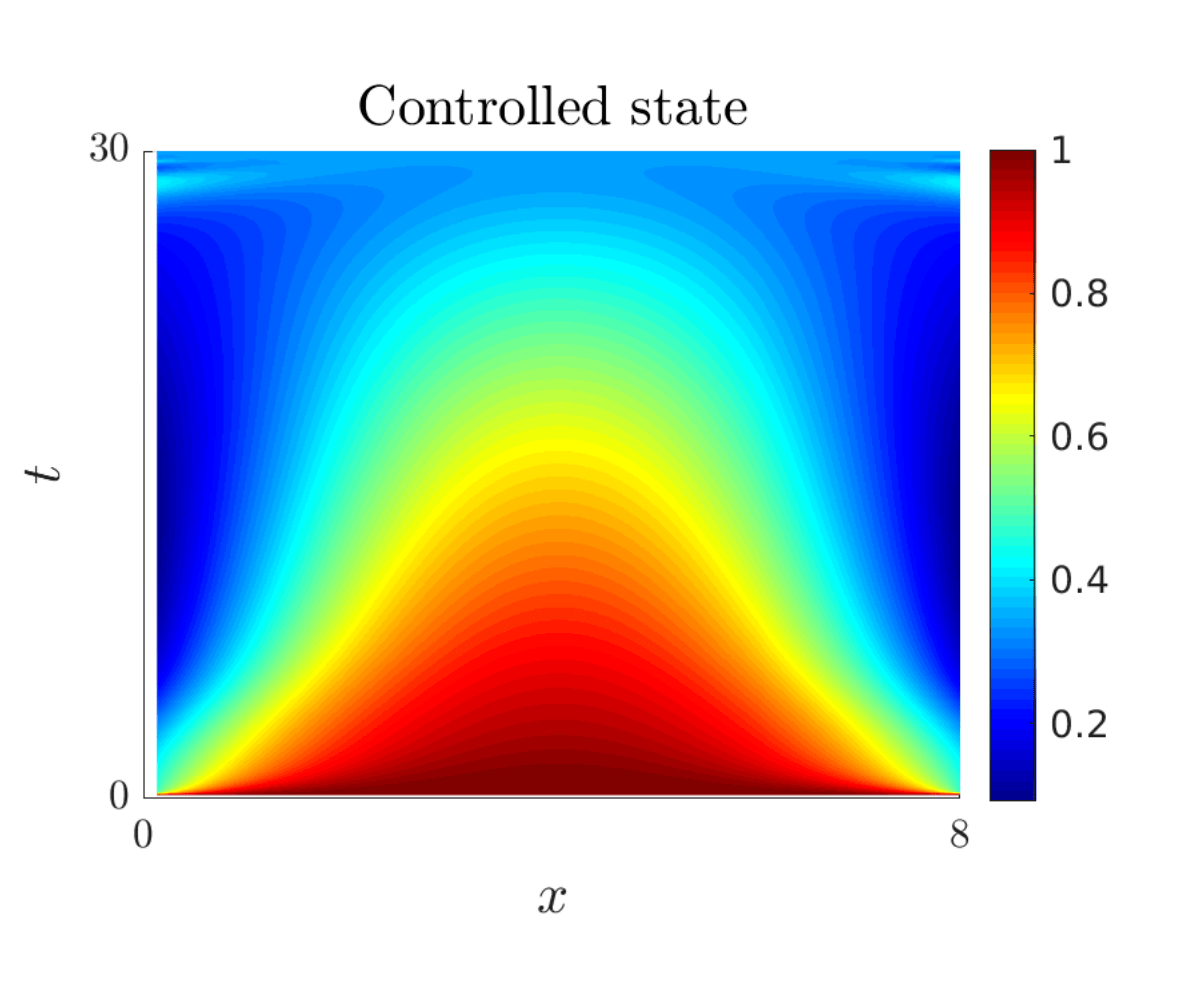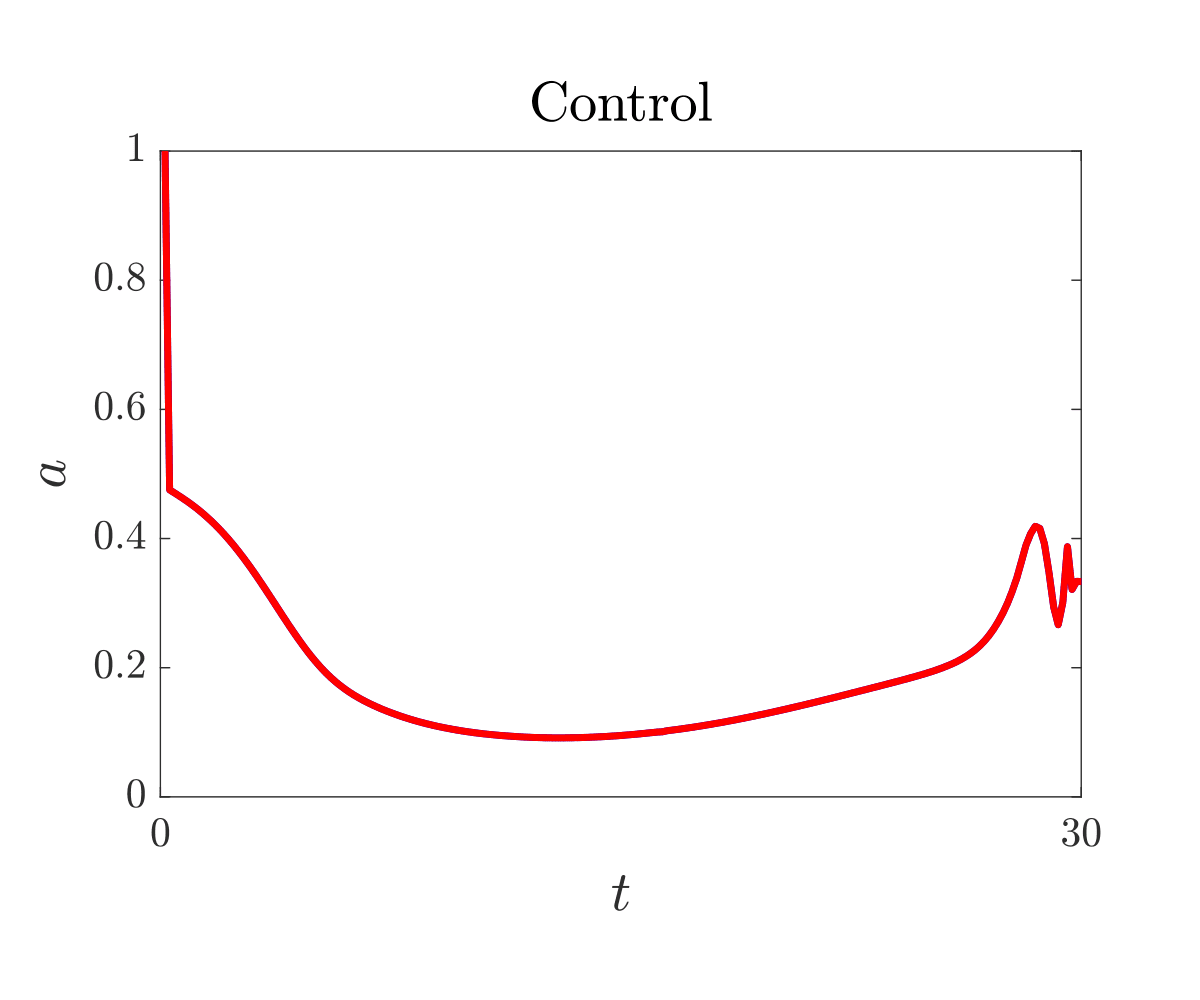Figure 1. At the left, the controlled state, at the right, the control function. $u_0=1$ and $u_1=0.33$.

Another important feature is the existence of a minimal controllability time. In Figure 2, $u_0=0$ and the target is $u_1=0.33$. From the theoretical perspective, if the time horizon is large enough we are always able to go from the initial state to the target. This is due to the existence of an admissible continuous path of steady states (Staircase post).

However, in Figure 2, one observes that the target $u_1=0.33$ has been not achieved. The reason is that $T$ is not large enough for the equation to be controllable.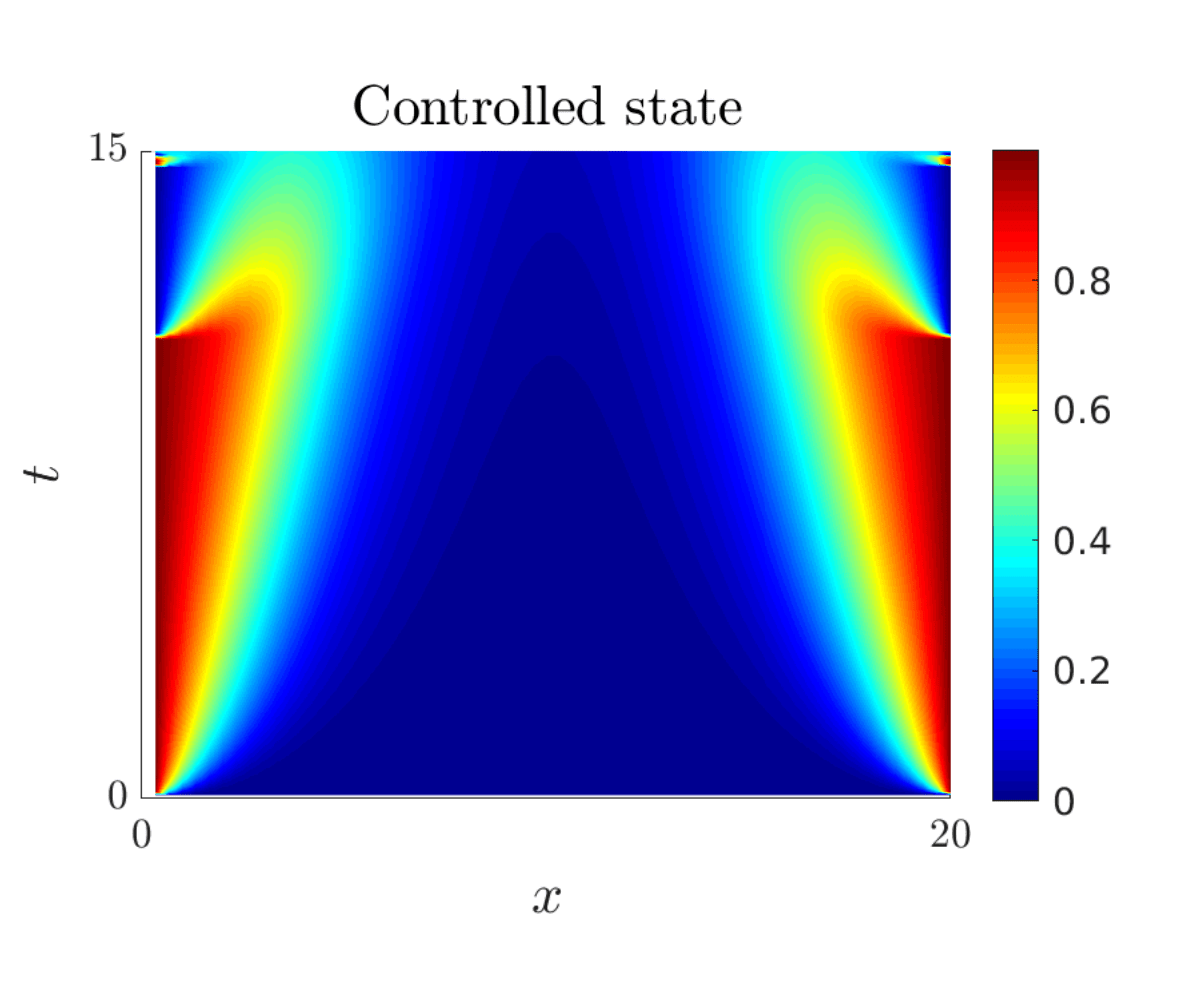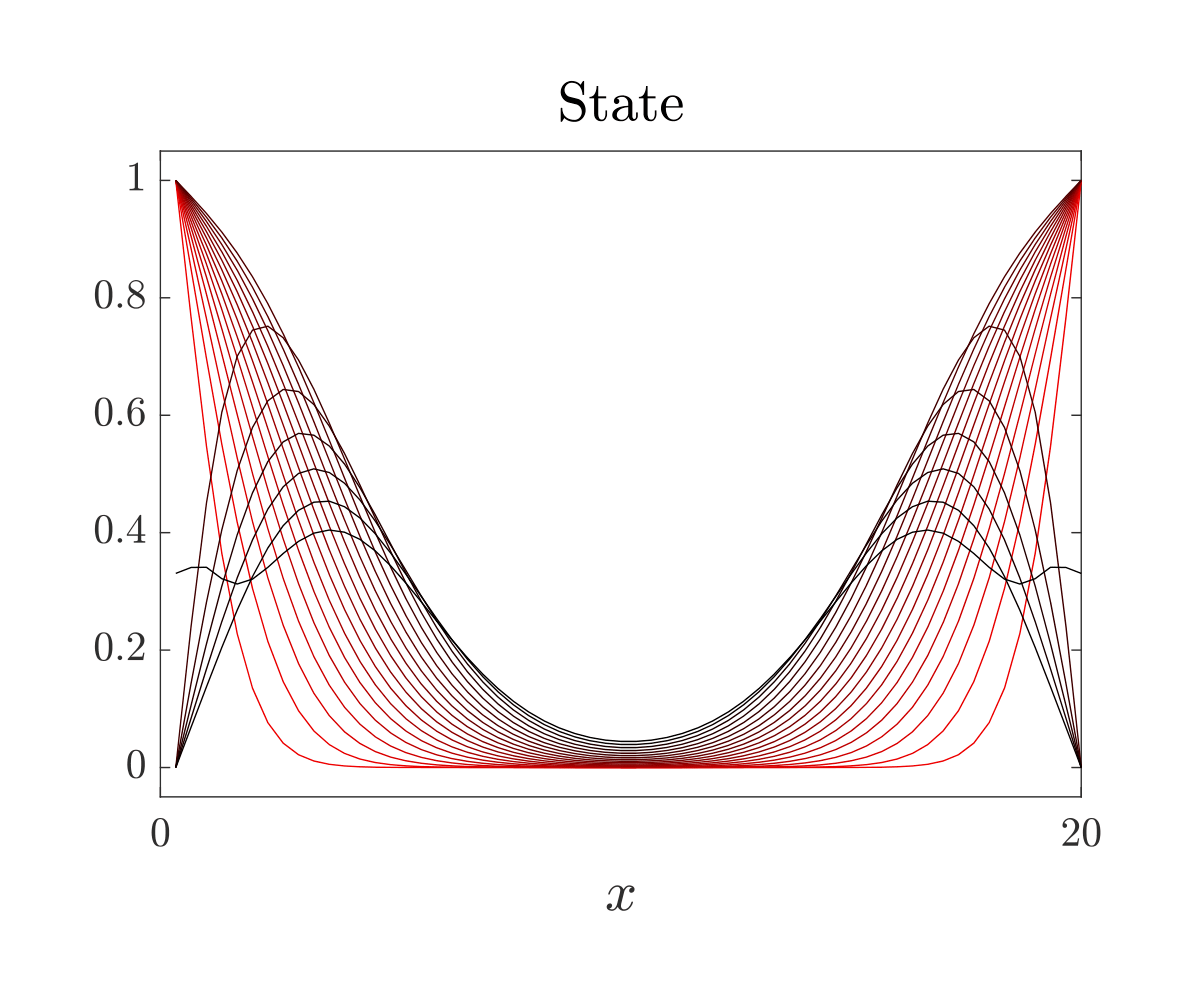Figure 2. At the left, the controlled state displayed in space-time, at the right, different snapshots of the state, darker curves are associated with larger times. $u_0=0$ and $u_1=0.33$ .

The other phenomenology already mentioned above and in the blog post Barriers is the lack of controllability due to the emergence of nontrivial solutions. In Figure 3, the initial state is $u_0=1$ and the target is $u_1=0$. We can observe how the state gets stuck to the barrier.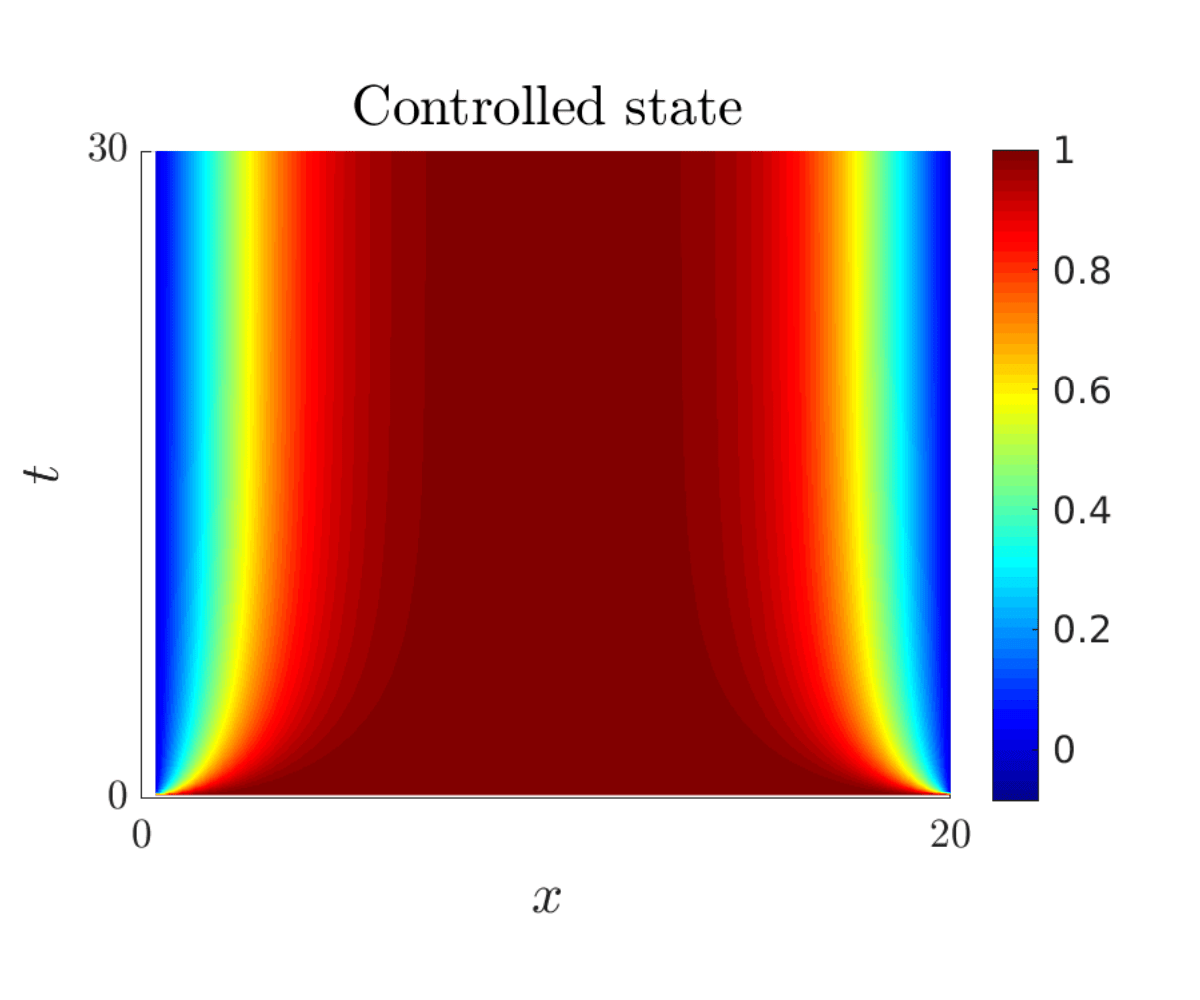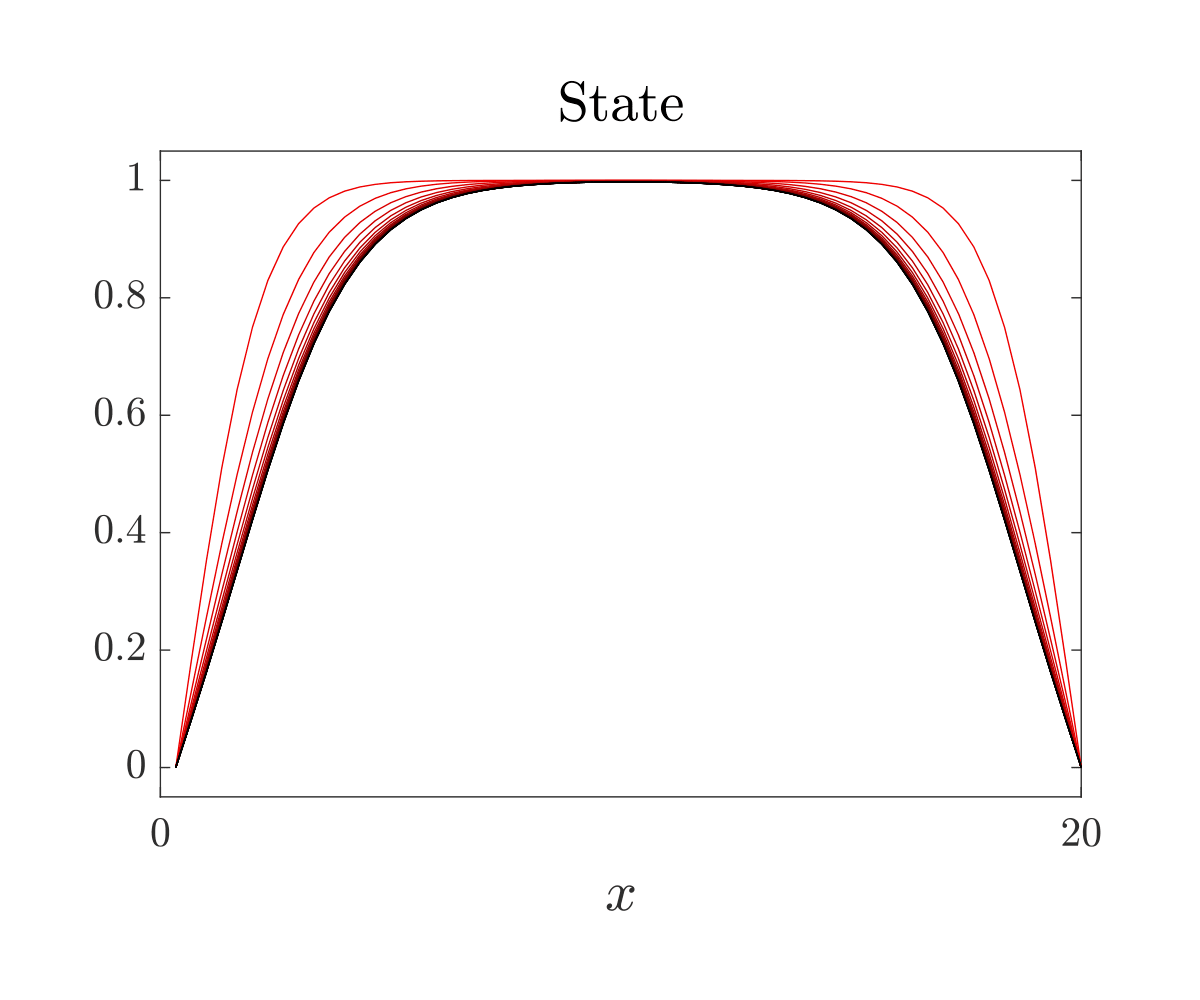Figure 3. At the left, the controlled state displayed in space-time, at the right, different snapshots of the state, darker curves are associated with larger times. $u_0=1$ and $u_1=0$ .

## Exploration

Now we turn our attention to the exploration of different control strategies.

### Quasistatic

In Figure 4, we seek to find a control that is quasistatic, for doing so we penalize the time derivative of the control in the discrete level and we add the restriction $u(x,T)=0.33$.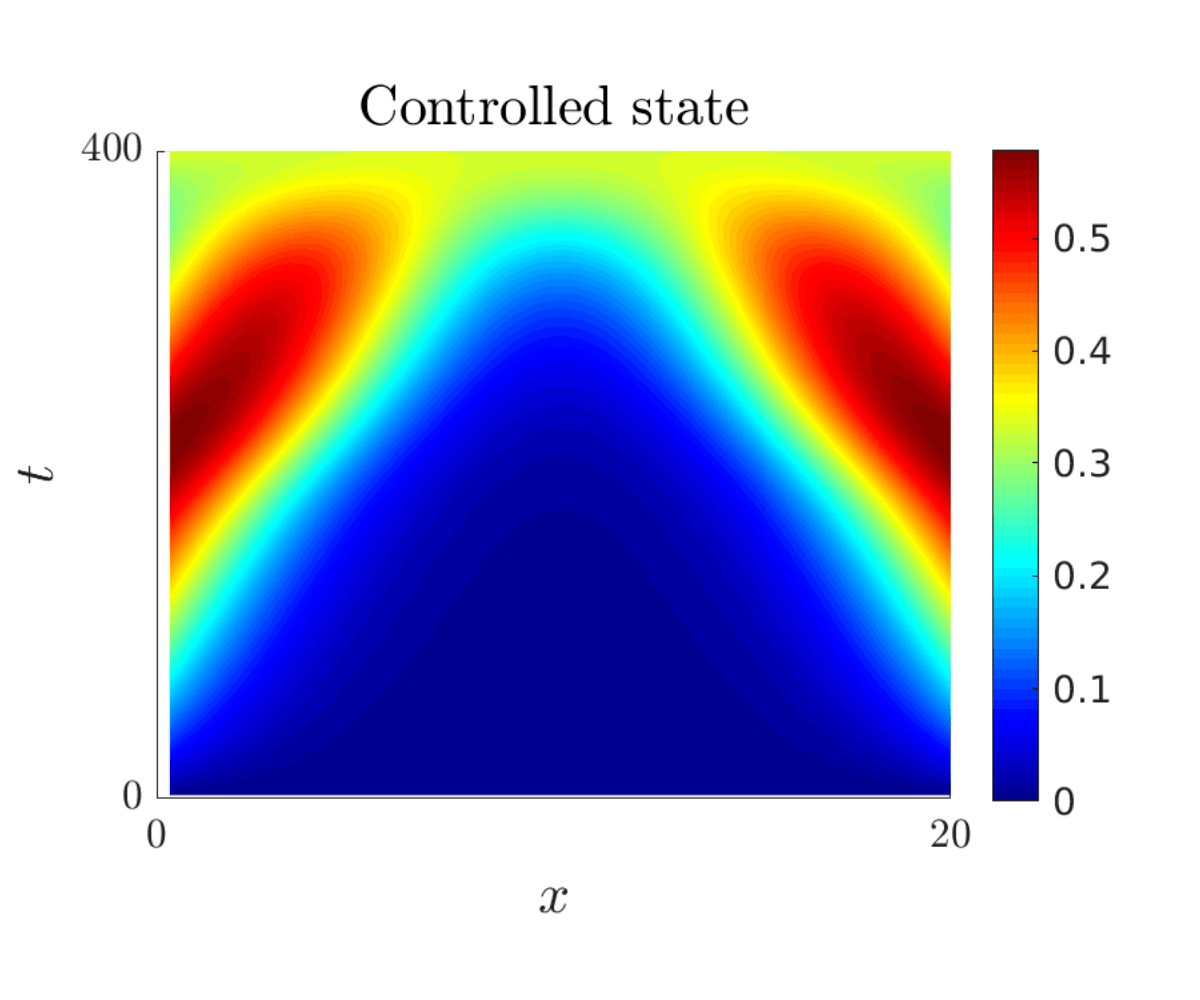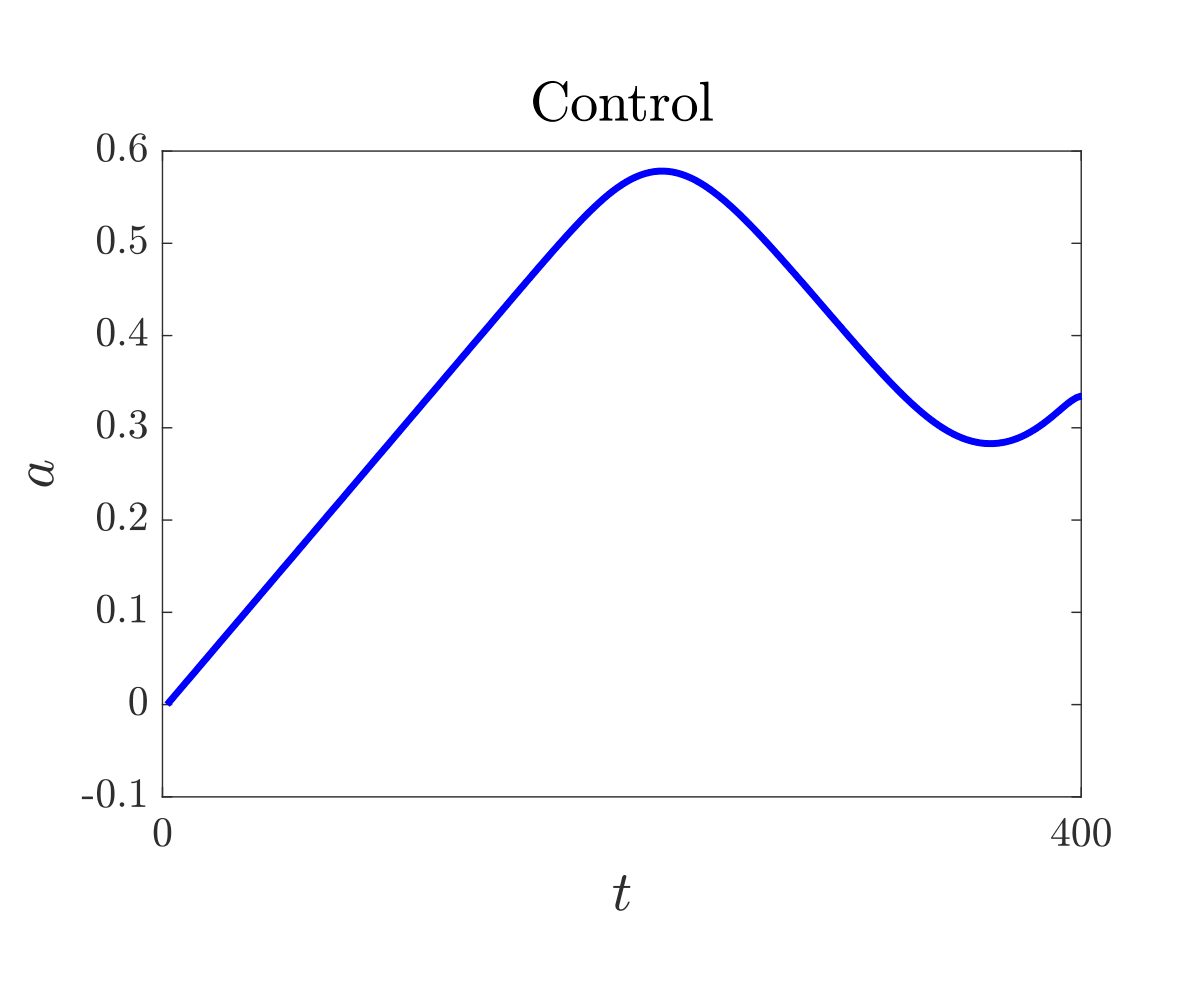Figure 4. At the left, the controlled state displayed in space-time, at the right, the control function. $u_0=0$ and $u_1=0.33$ .

# Minimal control time

In Figure 5, we compute the control in minimal time by minimizing the functional: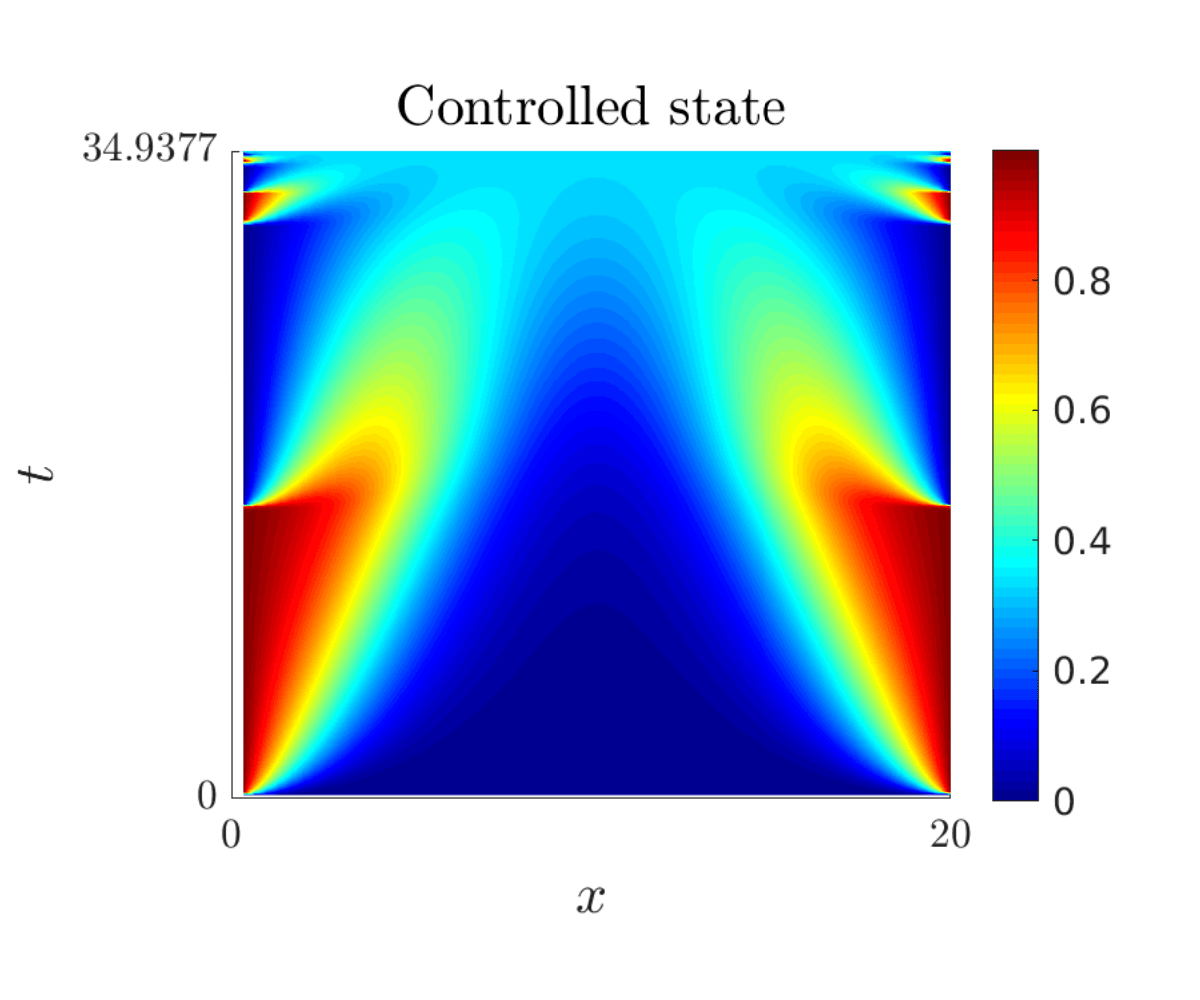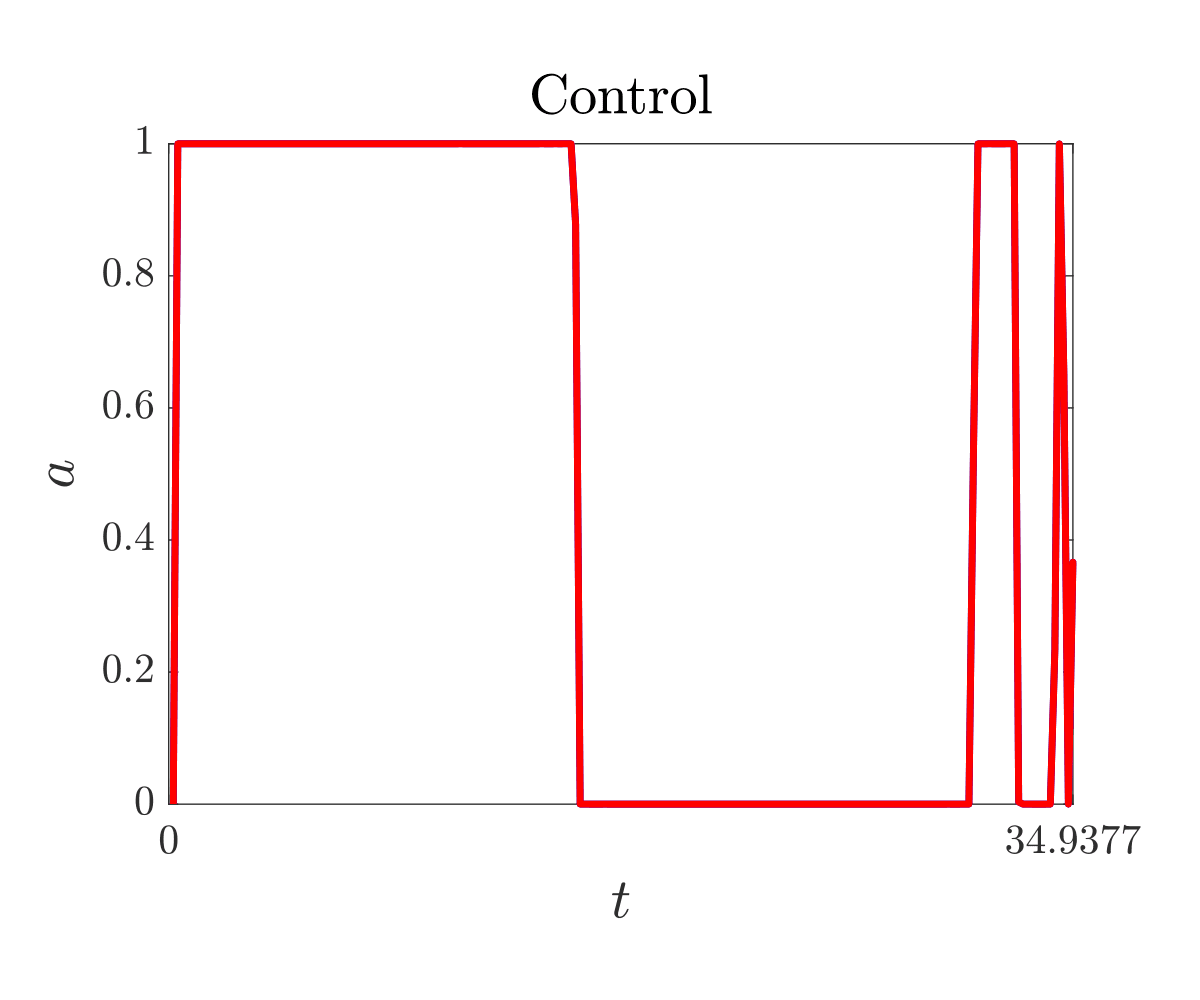Figure 5. At the left, the controlled state displayed in space-time, at the right, the control function. $u_0=0$ and $u_1=0.33$ .

Figure 5 points out that the control in minimal time has the bang-bang property.

### Minimal flow control

In Figure 6, we compute the control with minimal flow by minimizing the functional: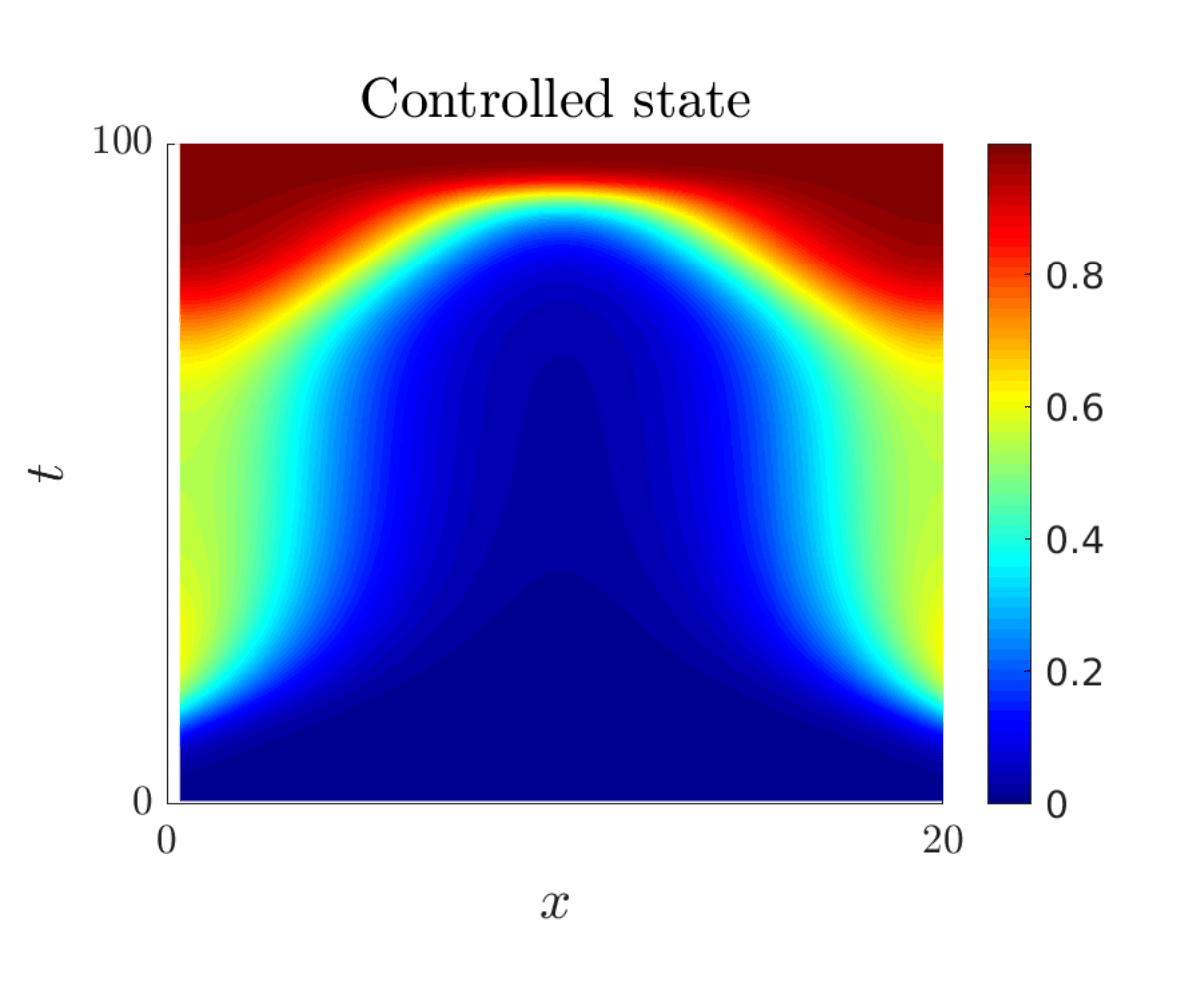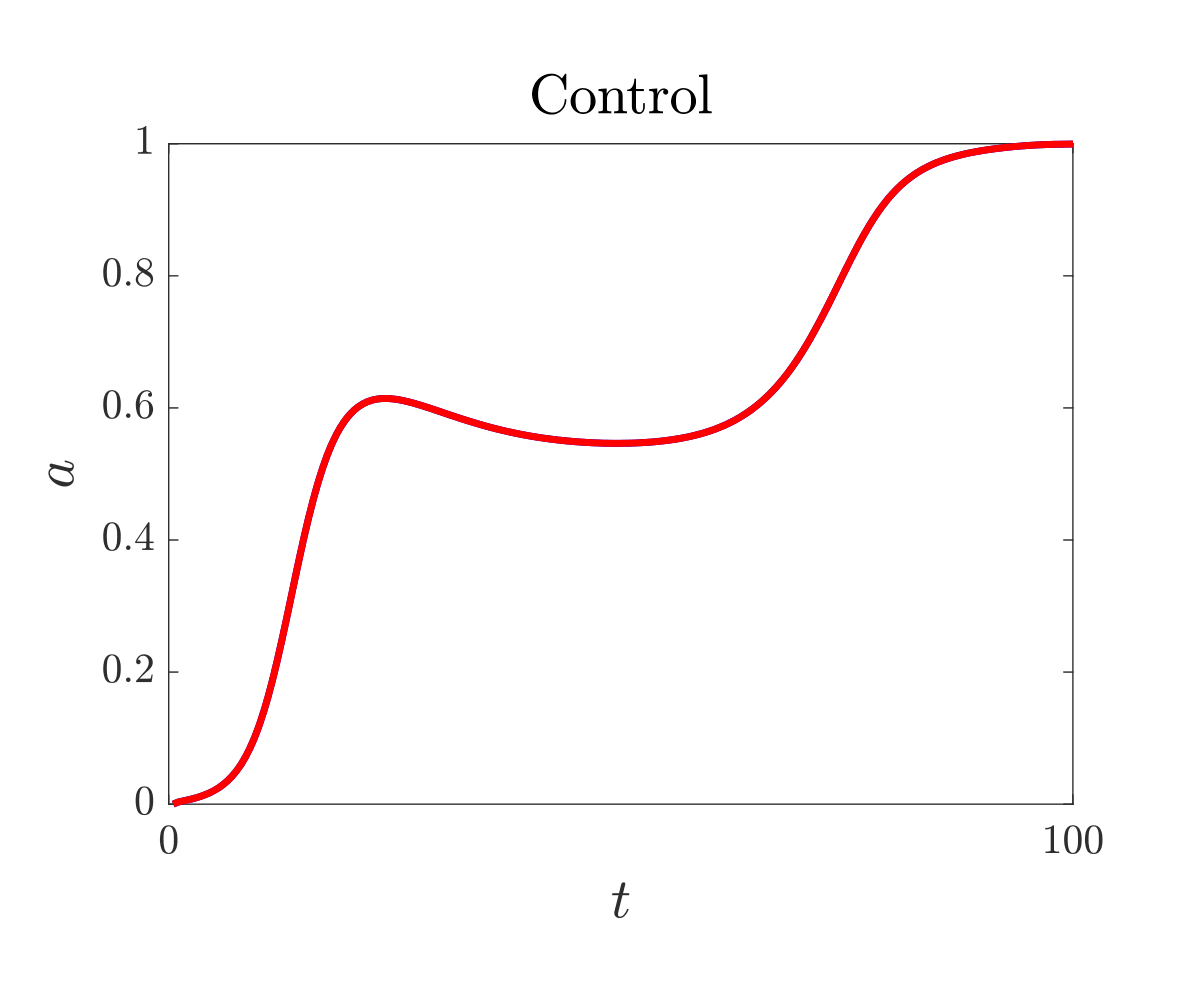Figure 6.

 D. Ruiz-Balet and E. Zuazua. Control of certain parabolic models from biology and social sciences. Preprint available at https://cmc.deusto.eus/domenec-ruiz-balet/.

 J.-M. Coron, E. Trélat, Global steady-state controllability of one-dimensional semilinear heat equa- tions, SIAM J. Control. Optim. 43 (2) (2004) 549–569.

 C. Pouchol, E. Trélat, E. Zuazua, Phase portrait control for 1d monostable and bistable reac tion–diffusion equations, Nonlinearity 32 (3) (2019) 884–909.

 R. Glowinski, J. L. Lions and J. He. “Exact and approximate controllability for distributed parameter systems: a numerical approach.” Encyclopedia of Mathematics and its Applications (2008).